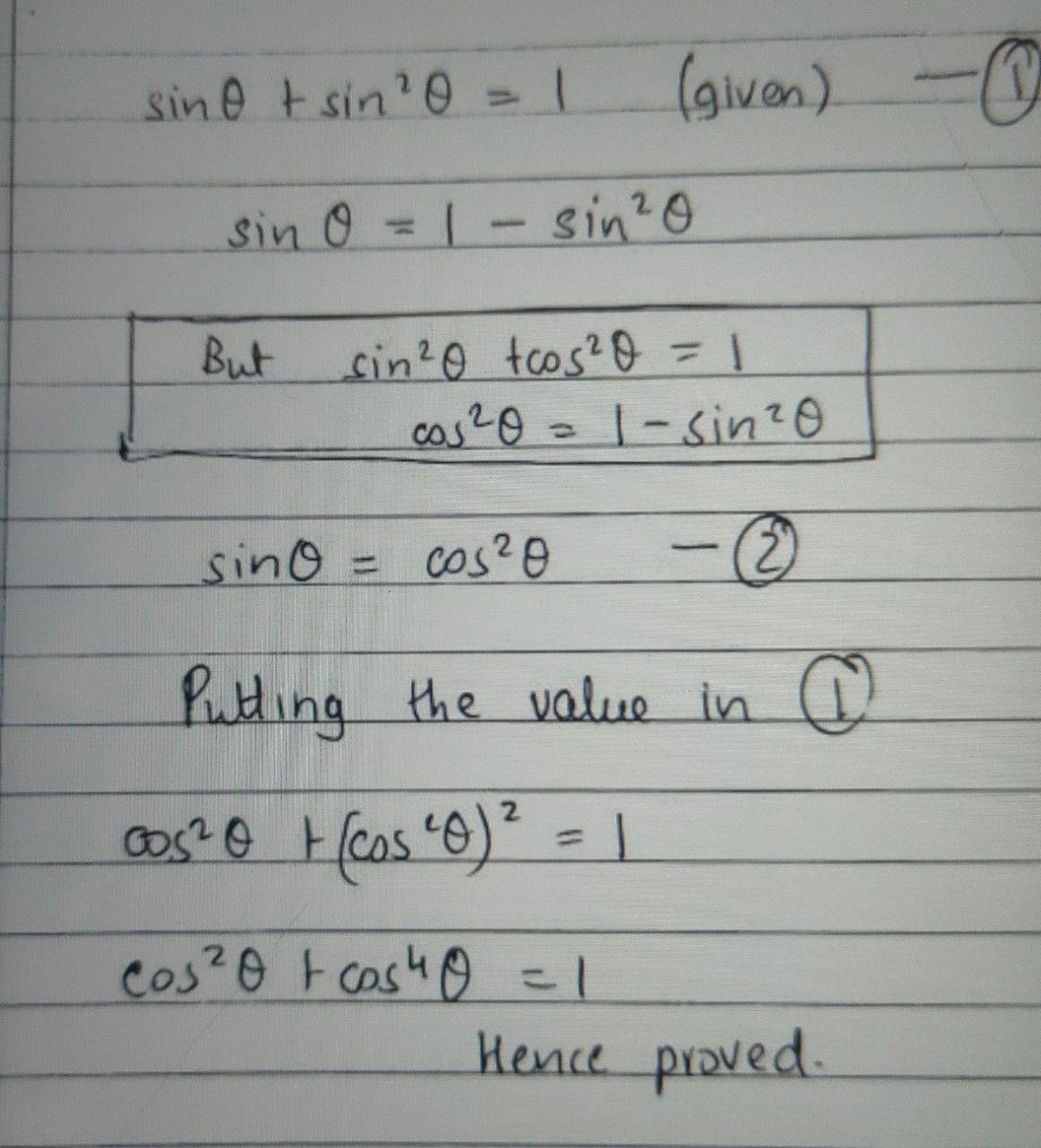Symbol
ProblemIf $sin$ $theta+cos$ $heta=1,$ then find the value of $si$ $2theta+cos$ $2$ 2theta sin $2$
Calculus
Search count: 117
SolutionQanda teacher - priyanka